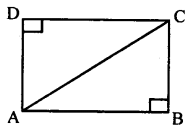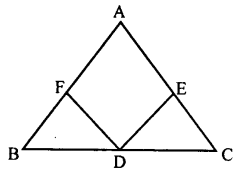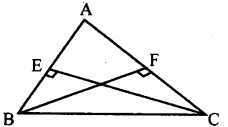# KSEEB Solutions for Class 8 Maths Chapter 11 Congruency of Triangles Ex 11.6

In this chapter, we provide KSEEB SSLC Class 8 Maths Chapter 11 Congruency of Triangles Ex 11.6 for English medium students, Which will very helpful for every student in their exams. Students can download the latest KSEEB SSLC Class 8 Maths Chapter 11 Congruency of Triangles Ex 11.6 pdf, free KSEEB SSLC Class 8 Maths Chapter 11 Congruency of Triangles Ex 11.6 pdf download. Now you will get step by step solution to each question.

### Karnataka State Syllabus Class 8 Maths Chapter 11 Congruency of Triangles Ex 11.6

Question 1.
Suppose ABCD is a rectangle. Using RHS theorem prove that the triangles ABC and ADC are congruent.In ∆ ABC and ∆ ADC
∠ABC = ∠ ADC = 90°
[Angles of a rectangle]
AC = AC [Common side]
AB = DC [Opposite side rectangle]
∴∆ ABC = ∆ ADC [RHS theorem]

Question 2.
Suppose ABC is a triangle and D is the midpoint of BC. Assume that the perpendiculars from D to AB and AC are of equal length. Prove that ABC is isosceles.DF ⊥ AB and DE ⊥ AC
In ∆BFD and ∆CED
∠BFD = ∠ CEP [= 90° by data]
BD = DC [D is the midpoint of BC]
DF = DE[data]
∴ ∆ BFD ≅ ∆CED [RHS theorem]
∴∠B =∠C [Corresponding angles]
∴AC = AB [Theorem 2]

Question 3.
Suppose ABC is a triangle in which BE and CF are respectively the perpendiculars to the sides AC and AB.
If BE = CF. Prove that triangle ABC is isosceles.In ∆ BFC and ∆ BEC
∠BFC = ∠BEC [= 90° data]
BC = BC [Common side]
CF = BE [data]
∴ ∆BFC ≅ ∆BEC [RHStheorem]
∠EBC = ∠FCB [Corresponding angle]
i. e., AB = AC
∴ ∆ ABC is isosceles

All Chapter KSEEB Solutions For Class 8 maths

—————————————————————————–

All Subject KSEEB Solutions For Class 8

*************************************************

I think you got complete solutions for this chapter. If You have any queries regarding this chapter, please comment on the below section our subject teacher will answer you. We tried our best to give complete solutions so you got good marks in your exam.

If these solutions have helped you, you can also share kseebsolutionsfor.com to your friends.

Best of Luck!!